# 2016广东高职高考数学试卷真题及答案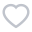289人已考
•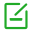卷面总分：150
•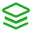试卷类型：历年真题
•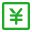测试费用： 免费
•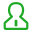关注人数：4085
•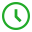作答时间：120分钟
•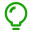答案：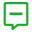试卷简介：
2016广东高职高考数学试卷真题及答案，本套试卷是2016广东高职高考数学真题，有答案。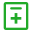试题类型：
• 单选题
• 填空题
• 解答题• 单选题
• 填空题
• 解答题
1 设集合 A={2,3, a}, B={1,4}, 且A ∩B ={4 }，则a = （ ）

A.1

B.2

C.3

D.4

2 函数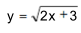的定义域是（ ）

A.（-∞,+∞）

B.[-3/2,+∞)

C.(-∞, -3/2]

D.( 0,+∞)

3 若a,b为实数，则 ' b=3' 是a( b-3)= 0的（ ）

A.充分非必要条件

B.必要非充分条件

C.充分必要条件

D.非充分必要条件

4 不等式 x^2-5x-6≤0 的解集是（ ）

A.{x∣-2≤x≤3}

B.{x∣-1≤x≤6}

C.{x∣-6≤x≤1}

D.{x∣x≤-1或x≥6}

5 下列函数在其定义域内单调递增的是（ ）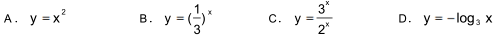A.A

B.B

C.C

D.D

6 函数 y=cos( π/2 - x )在区间[π/3, 5π/6]上的最大值是（ ）

A.1/2

B.√2/2

C.√3/2

D.1

7 设向量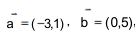则 ∣a- b∣=（ ）

A.1

B.3

C.4

D.5

8 在等比数列{an} 中，已知a3=7,a6=56，则该等比数列的公比是（ ）

A.2

B.3

C.4

D.8

9 函数y=(sin2x-cos2x)^2的中最小正周期是（ ）

A.Π/2

B.Π

C.2Π

D.4Π

10 已知 f(x) 为偶函数，且 y=f(x)的图像经过点 (2 , -5 ) ，则下列等式恒成立的是（ ）

A.f(-5)=2

B.f(-5)=-2

C.f(-2)=5

D.f(-2)=-5